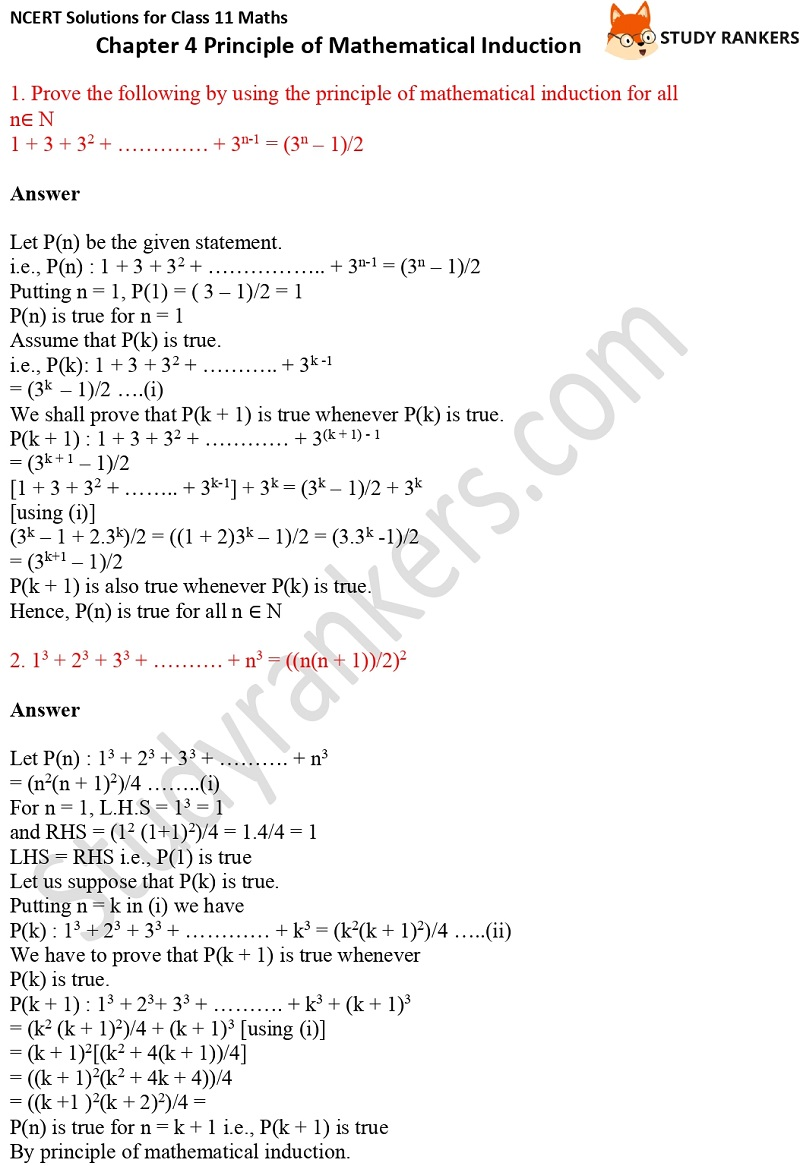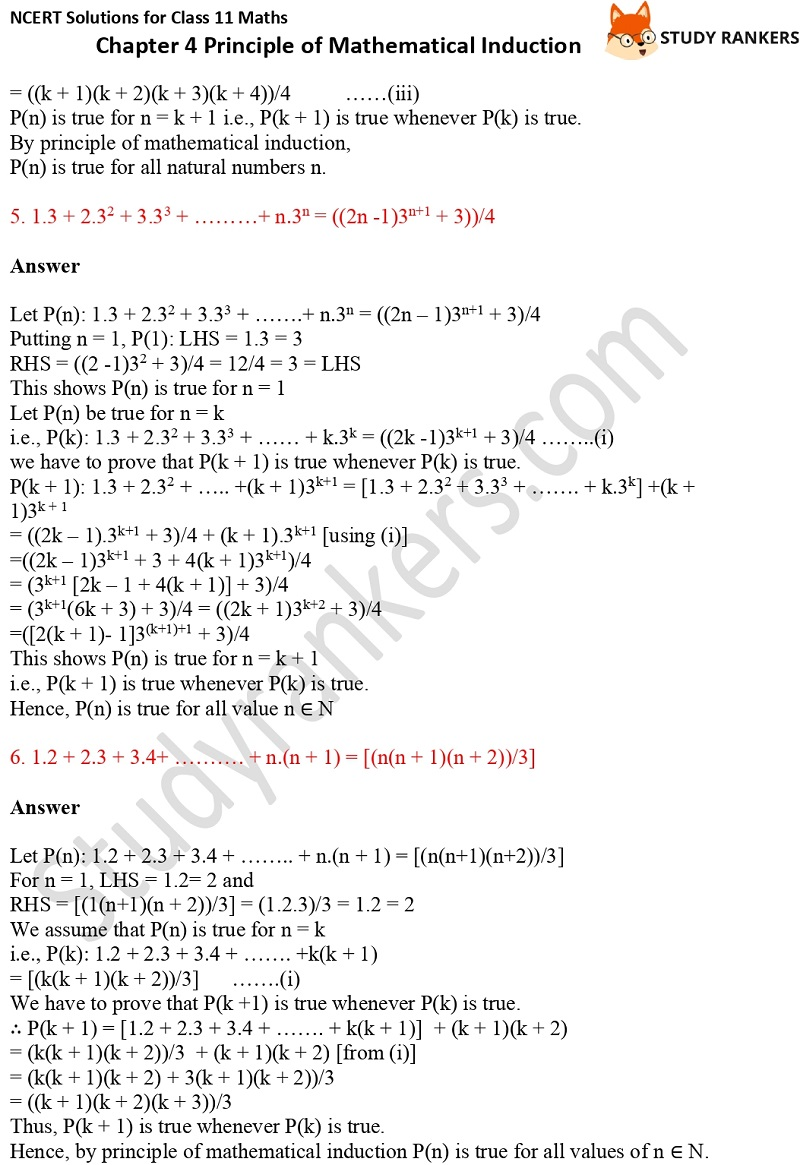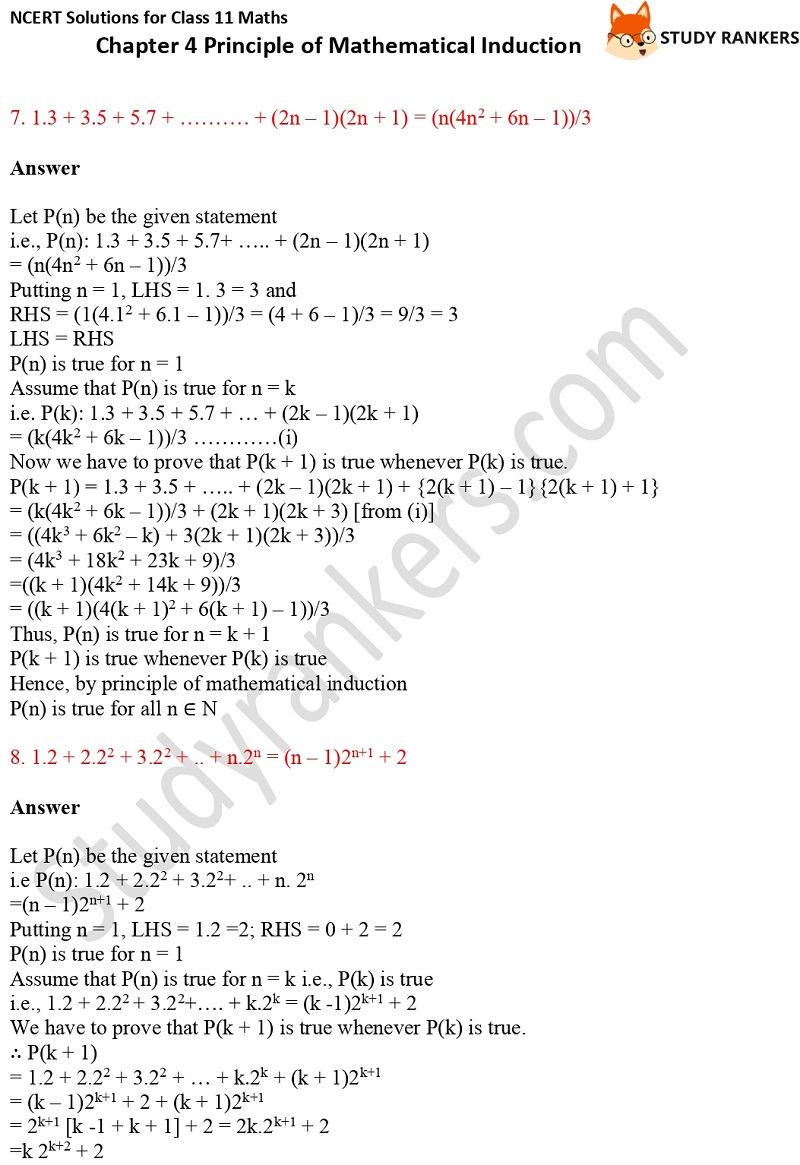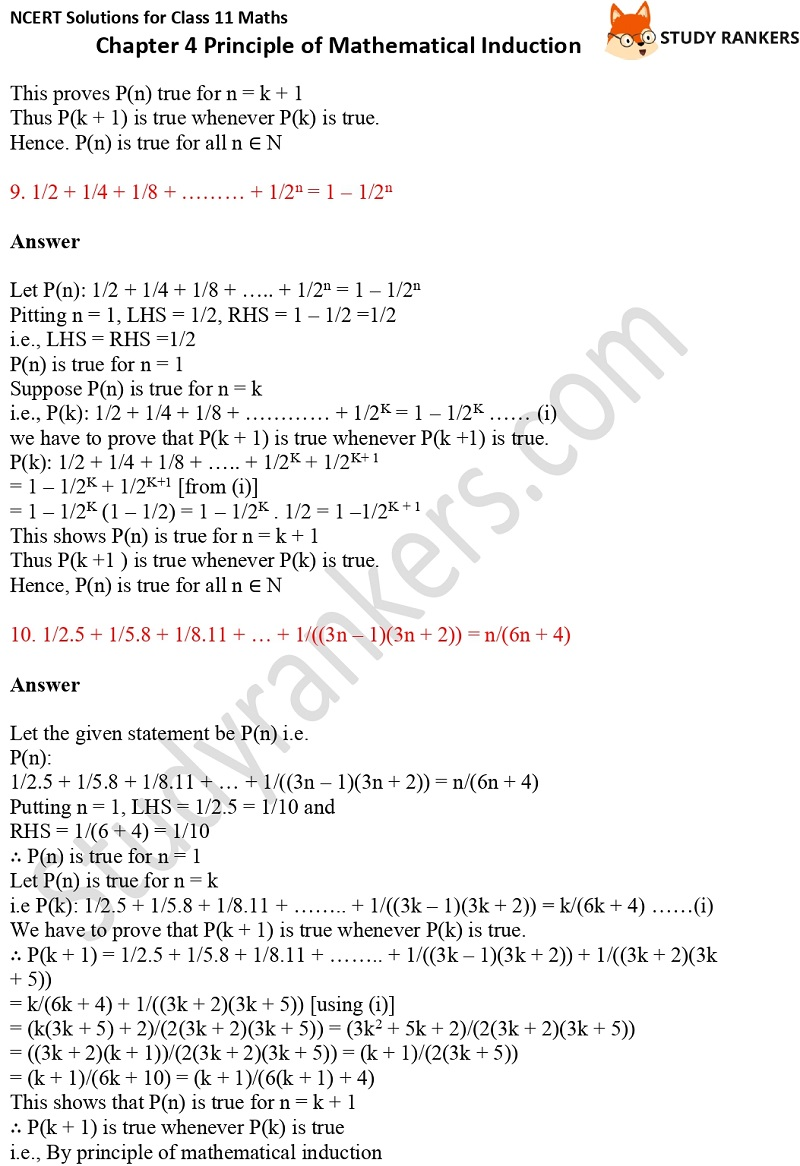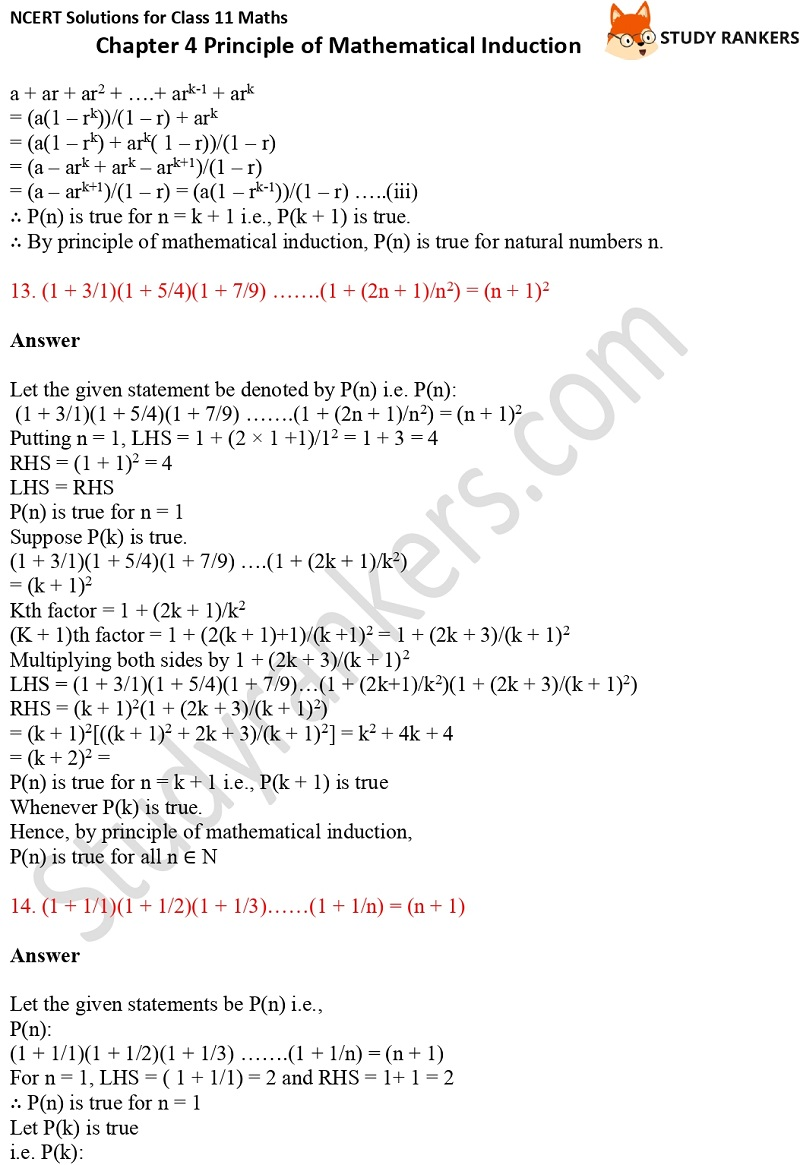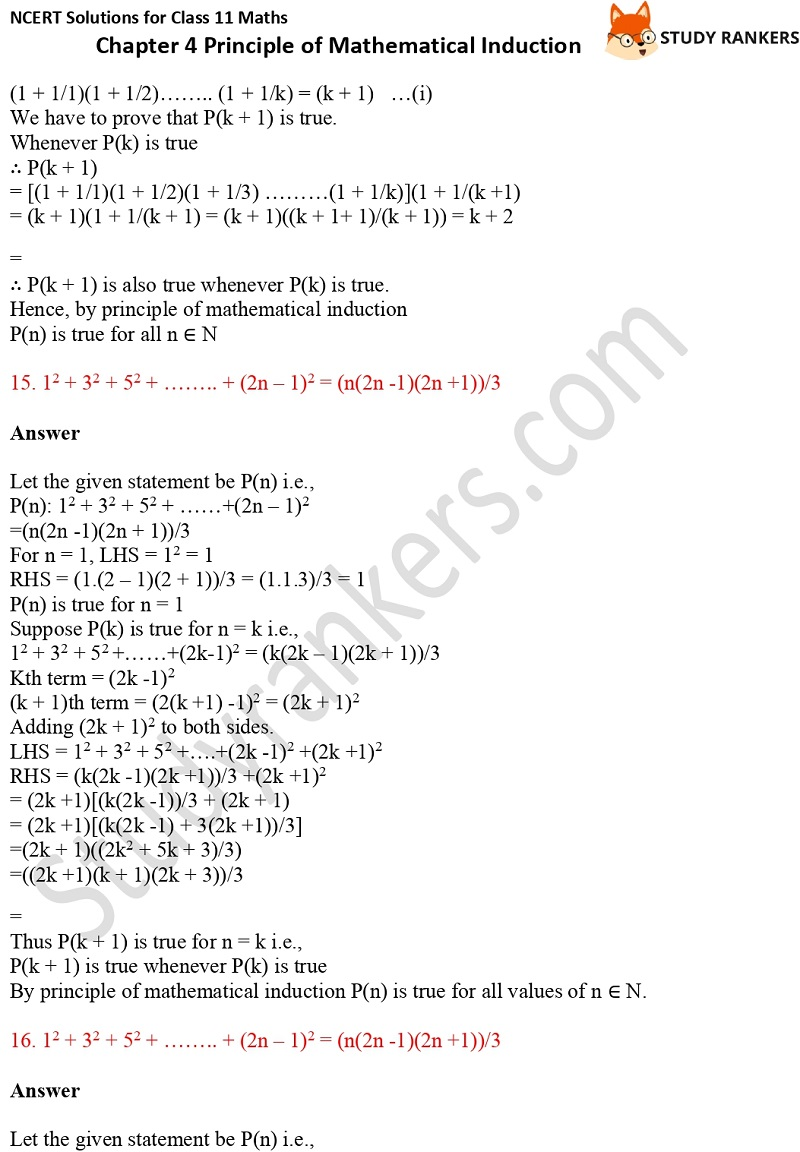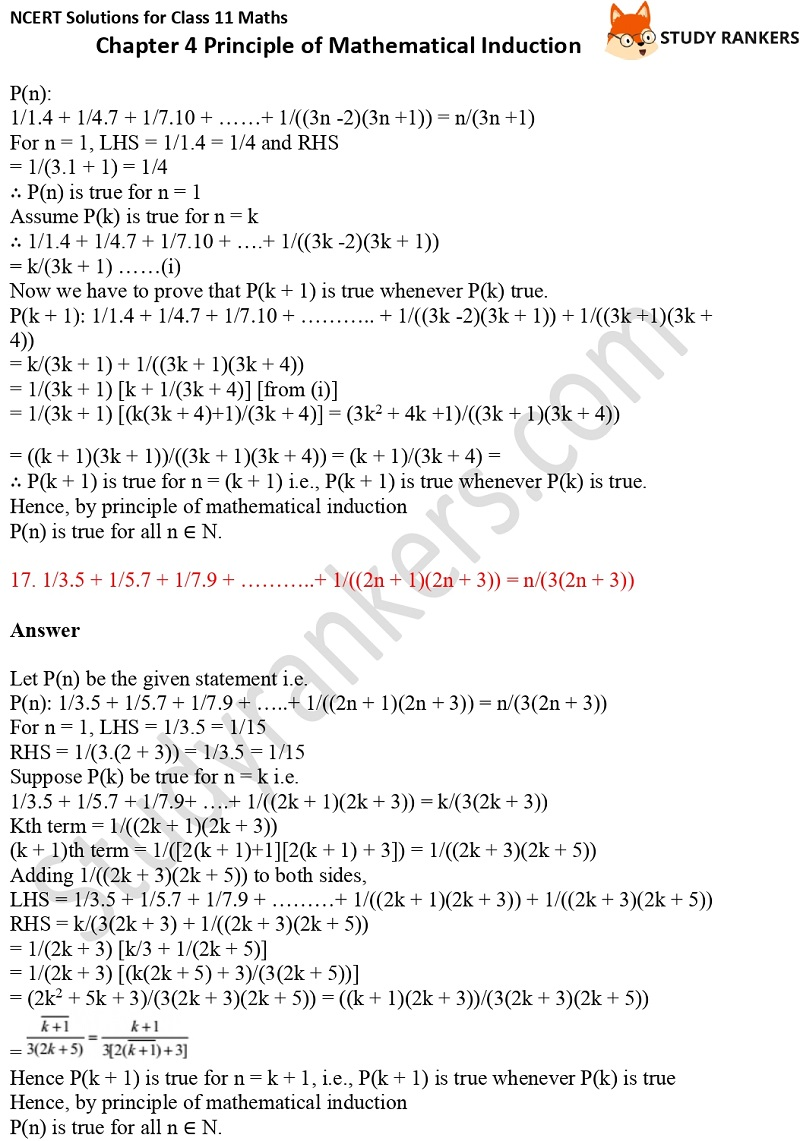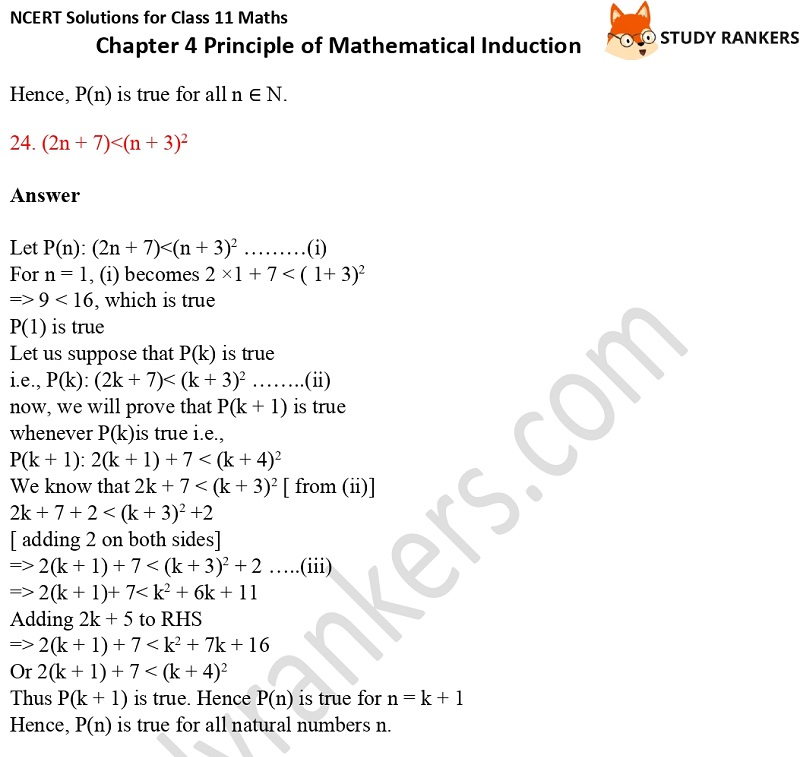>

# NCERT Solutions for Class 11 Maths Chapter 4 Principle of Mathematical Induction

Chapter 4 Principle of Mathematical Induction Class 11 Mathematics NCERT Solutions can be useful in preparing answers by taking help. These NCERT Solutions for Class 11 will help you in attaining better marks in the examinations. It will be useful in clearing your doubts and completing homework in no time.

In Exercise 4.1, you have to prove the given by using the principle of mathematical induction for all n∈ N.